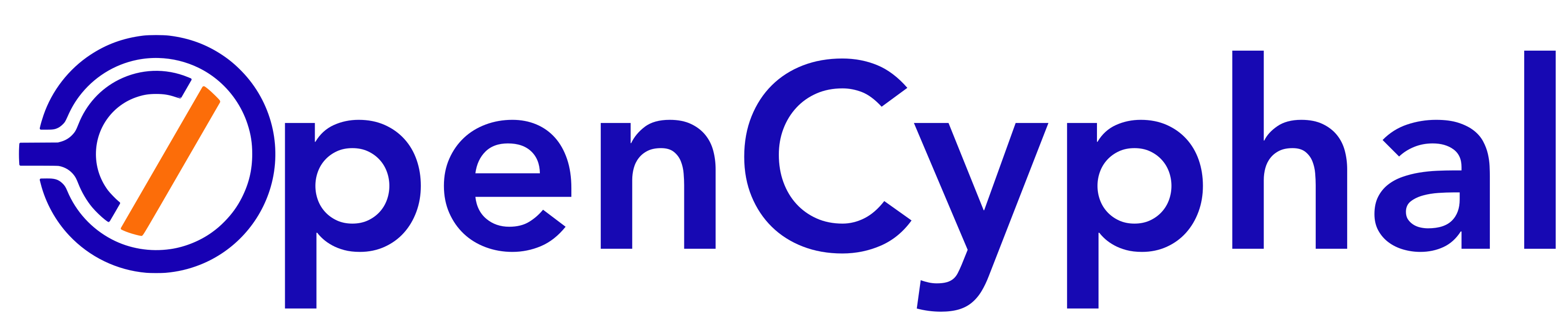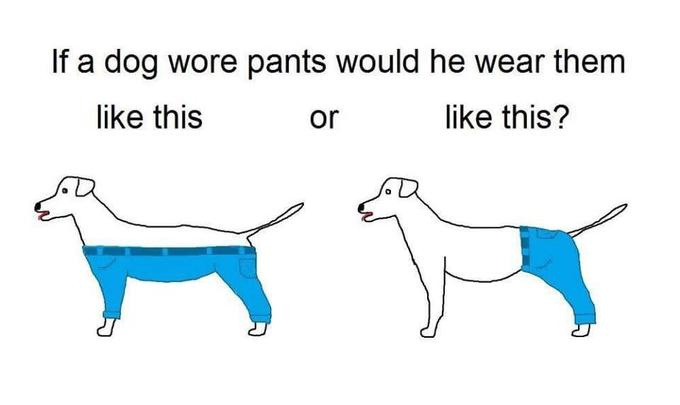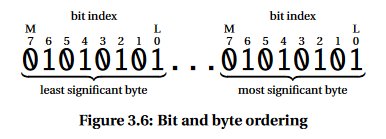# Big-endian vs. little-endian in the context of bit-level encoding

## From Scott

Sticking with the simple example for now:

Given dsdl/VarArray.1.0.uavcan as

``````uint8 [<=16] data
``````
``````import pyuavcan, dsdl
data = list(range(254,254-14,-1))
print('input : {}'.format(data))
``````

input : [254, 253, 252, 251, 250, 249, 248, 247, 246, 245, 244, 243, 242, 241]

``````var_bits = pyuavcan.dsdl.serialize(dsdl.VarArray_1_0(data))
bit_string = next(map(lambda x: bytes(x).hex(), var_bits))
print(bit_string)
bytes_array = []
for i in range(0, len(bit_string), 2):
bytes_array.append(int(bit_string[i:i+2], base=16))

# raw value (what goes on the wire in the current implementation)
print('hex: {}'.format(['{:02X}'.format(x) for x in bytes_array]))
print('dec: {}'.format(['{}'.format(x) for x in bytes_array]))
77f7efe7dfd7cfc7bfb7afa79f9788
``````

hex: [‘77’, ‘F7’, ‘EF’, ‘E7’, ‘DF’, ‘D7’, ‘CF’, ‘C7’, ‘BF’, ‘B7’, ‘AF’, ‘A7’, ‘9F’, ‘97’, ‘88’]
dec: [‘119’, ‘247’, ‘239’, ‘231’, ‘223’, ‘215’, ‘207’, ‘199’, ‘191’, ‘183’, ‘175’, ‘167’, ‘159’, ‘151’, ‘136’]

``````# This is what is implemented
​
data_reconstructed = []
​
# First 5 bits are the fill
fill = (bytes_array & 0xF0) >> 3
print('fill should be {} is {}'.format(len(data), fill))
​
# This means the fill field starts at bit 3 which violates LSB rules.
for i in range(1, 15):
# Each element is then made out of the lowest 3 bits of the previous byte
# and (OR) the last 5 bits of next byte. This means the next element begins at
# bit 3 which, again, violates LSB rules.
bte = (bytes_array[i - 1] & 0x7) << 5 | ((bytes_array[i] & 0xF8) >> 3)
data_reconstructed.append(bte)
print(data_reconstructed)
``````

fill should be 14 is 14
[254, 253, 252, 251, 250, 249, 248, 247, 246, 245, 244, 243, 242, 241]

``````# This is what we expect
​
data_reconstructed = []
​
# The fill begins at bit 1 per LSB format
fill = bytes_array & 0x1F
print('fill should be {} is {}'.format(len(data), fill))
​
for i in range(0, 14):
# Each element in the array begins at bit 5 of a byte and then continues
# from bit 0 in the next byte.
bte = ((bytes_array[i+1] & 0x7F) << 3) | ((bytes_array[i] & 0xE0) >> 5)
data_reconstructed.append(bte)
print(data_reconstructed)
``````

fill should be 14 is 23
[955, 895, 831, 767, 702, 638, 574, 510, 445, 381, 317, 253, 188, 68]

## The Intel format (little-endian rules):

1. Bytes are ordered LSB
2. Bits are ordered LSB
3. Parts of the bytes are ordered LSB – UAVCAN has a bug in this rule by placing fractional byte fields in Motorola format (big-endian). We followed rules 1 and 2 above (so bits inside those fractional byte fields are LSB), but we wrongly selected which part of byte to occupy by the fractional byte field (and the rest of following data).

Our experience has been that this implementation is incompatible with all known CAN databases and vendor tools for processing frames.

The intention of the messages I posted above was to show that the current implementations (PyUAVCAN, Libuavcan, Libcanard) are spec-compliant. I did not mean to imply that the currently specified behavior is optimal.

You are saying that the culprit is here:

Do we want to change it? The affected sections of the specification are:

• 3.7.1.2 Bit and byte ordering
• 3.7.3.1 General principles
• 3.7.5 Composite types
• 4.2.3 Examples

I believe I may have taken on faith something that I’m not able to personally verify. Specifically, my colleague asserted this part of my last post:

I’m not able to find that LSB dictates any bit order. This seems to be left to the realm of network transports and the only thing I’ve found for CAN are:

1. Vector tools consider MSb (Most-Significant-bit) to be “inverted” but also supports this format without difficulty (according to http://vi-firmware.openxcplatform.com/en/master/config/bit-numbering.html)
2. Most CAN peripherals integrated with LSB architectures expect MSb bit-ordering (based on my personal experience)

Given these two facts, it would seem changing this part of the specification is a bad idea. What we need to run to ground (and perhaps this is something I need to dive a bit deeper into with the tools vendors that have reported difficulties with our unaligned data) is, why are generic tools having trouble comprehending UAVCAN data payloads only when they are unaligned? I wonder if Kvaser could shed some light onto this?

Also, the diagram 3.7.1.2 is very misleading. Being someone that reads diagrams first and text second I assumed that you were specifying LSb since bit-number 0 is almost always shown as the LSb graphically (see any binary calculator, ever). I believe you should invert the bit numbering in this diagram and the associated text such that “the most significant bit is considered first (‘8th bit’, ‘bit 7’, or ‘the first bit received’)” and relabel the diagram to start at 7 with the label being “bit numbering”. We should additionally show a wire-level diagram that shows that “bit 7” is the first received for a byte (i.e. Most-Significant bit first) and perhaps point out that the CAN specification itself required MSb in the data field.

I think that as long as we stay with least-significant byte first (which is important for best compatibility with modern CPUs), everything else is a matter of convention so it can be changed freely. I will be standing by for the results of your analysis of bit-level compatibility with other solutions out there.

The bit numbering in the diagram 3.6 is intended to demonstrate how to arrange subsequent fields within a byte. We say that the most significant bit is the first one, index 0. What it means is that if we have a structure like this:

``````uint2 a
uint3 b
uint1 c
``````

It goes into a byte like this (one symbol – one bit here; x – padding):

``````aabbbcxx
``````

``````xxcbbbaa
``````

If you think it should be shown on the diagram in a more clear way, could you please open a PR in the spec repo?

FWIW, GCC and Clang on AMD64 use little-endian bit order:

``````#include <stdio.h>

struct A
{
unsigned a : 2;
unsigned b : 3;
unsigned c : 1;
unsigned d : 16;
};

union U
{
unsigned bits;
struct A a;
};

int main()
{
union U u = {0};
u.a.a = 3;
u.a.c = 1;
u.a.d = 0b1111110011001100;
printf("%x\n", (unsigned)u.bits);
return 0;
}
``````

Outputs 3f3323 which is 0b1111110011001100100011, which is d=1111110011001100 c=1 b=000 a=11.

Although, in Python, `numpy.packbits()` (and its counterpart `numpy.unpackbits()`) defaults to the big-endian bit order.

CANopen is bit-little-endian:

Comparison:

``````# dsdl/Test.1.0.uavcan
uint10 value
``````
``````\$ uvc dsdl-gen-pkg dsdl_src/dsdl/
\$ python3
>>> import pyuavcan, dsdl
>>> [bytes(x) for x in pyuavcan.dsdl.serialize(dsdl.Test_1_0(0x21C))]
[b'\x1c\x80']  # CANopen would have yielded b'\x1c\x02'
``````

One issue with the little-endian bit order is that it is poorly compatible with the implicit prefix alignment. By “implicit prefix alignment” I mean this: Proposed change to variable-length arrays, note that it is applicable not only to arrays but to unions also.

Suppose we have a definition containing a variable-length array like this:

``````void5
uint8[<8] a
``````

Or a definition containing a union of 8 fields:

``````void5
MyUnion.1.0 a
``````

Denote the void bits `v`, the implicit prefix bits `i`, and the value bits `a`. The current, big-endian rule results in the following layout:

``````vvvvviii aaaaaaaa ...
``````

Whereas the proposed little-endian rule results in the following layout:

``````iiivvvvv aaaaaaaa ...
``````

Observe that only the former (i.e., current format) is equivalent to:

``````iiiiiiii aaaaaaaa ...
``````

Only the current format allows implementations to substitute a pattern like `voidX + [u]intY` with `[u]int(X+Y)`.

This is not a major advantage, obviously, but the gains of the little-endian format are not high either.

As a reference, here is an implementation of the little-endian format in PyUAVCAN: https://github.com/UAVCAN/pyuavcan/pull/99

But in that section (7.1.3.2) it states:

Octet 1 is transmitted first and octet k is transmitted last. Hence the bit sequence is transferred as follows across the network:

b7, b6, …, b0, b15, …, b8, …

So we do see that CANopen is little-endian for bytes but if bit 7 is first in the first octet and bit 15 is first in the second octet how does it follow that CANopen is little-endian for bits?

In the example `b = b0 .. b9 = 0011 1000` this means the least-significant-byte (1st octet: 0x1C) would be transferred as such:

``````+--------------------------------------------+
| bit number | 7 | 6 | 5 | 4 | 3 | 2 | 1 | 0 |
| value      | 0 | 0 | 0 | 1 | 1 | 1 | 0 | 0 |
+--------------------------------------------+
``````

The most-significant-bit was transferred first here.

Let’s pause and first be sure we’re on the same page here to reduce ongoing confusion.

Yeah, we are confusing transfer syntax and serialization. We don’t care about the transfer syntax – it’s the domain of the transport layer. What we care about is how do we implement field boundaries when they do not match with byte boundaries.

A field whose boundary does not match with a byte boundary has to occupy only a fraction of the byte. The question is: should the fraction be aligned left or right?We have to define an ordering rule for bits within a byte. There are two sensible possibilities:

• Big-endian is when the most significant bit is considered to be first.
• Little-endian is when the least significant bit is considered to be first.

Suppose we have a field that takes three bits of a byte; let’s label its bits `f`, and the unoccupied bits would be `x`. Then, in the case of the big-endian format, the byte will be divided up as follows (MSB on the left, LSB on the right):

bit number 0 1 2 3 4 5 6 7
value f f f x x x x x

And the little-endian would be (same display: MSB on the left, LSB on the right):

bit number 7 6 5 4 3 2 1 0
value x x x x x f f f

Which bit is transmitted first is absolutely irrelevant.

Kent Lennartsson got back to me with a suggestion to “make as few changes between two versions as possible”, which translates into big-endian. He wrote other things on the subject as well but they do not relate directly to the discussion so I am omitting that.

Sergey said at the dev call that he is concerned about the fact that a byte-boundary-aligned number that takes n<8 bits will be represented on the wire as if it were shifted left by (8-n). In other words, suppose we have `uint3 x`, x=1, then a serialized representation would be (1<<(8-3)) = 32. Then (x+1) would be (2<<(8-3)) = 64, (x+2) → 96, etc.

I am not entirely against the little-endian format but my little experiment with PyUAVCAN (see PR 99, linked earlier) seems to indicate that it doesn’t really make things much more approachable. Whichever way you turn it, unaligned fields are a disaster and you can’t do anything about it.

This is what we propose:

Note that the convention used here is that bits are placed on the bus starting on the left and reading to the right. I.e: b7, b6, …, b0. This example demonstrates Least-Significant-Byte ordering with Most-Significant-Bit ordering.

Our experience is this convention maximizes compatibility with industry standard CAN databases offered by companies like Kvaser or Peak Systems.

Well. No wonder. This is the CANopen format. I don’t understand two things:

the convention used here is that bits are placed on the bus starting on the left and reading to the right

Where does the bus come into this discussion? Why do we care how the bits are transmitted? If my very special bus transmits even bits first, odd bits later, how and why does it affect the serialization format?

This example demonstrates Least-Significant-Byte ordering with Most-Significant-Bit ordering.

If we were to use the terminology I proposed in the previous post, the ordering would be least-significant-bit-first, because we align sub-byte fields on the right first (i.e., towards the LSB). Do you think the terminology is incorrect? Maybe we should just avoid saying things like MSB/LSB, seeing as we are not transmitting anything here, but merely smudging bits around?

Edit: also, how important the compatibility with COTS tools is, from your experience, on a scale from 1 to 5?

0004

Actually, it’s rather intuitive. It’s obvious but for some reason, I did not see it. In the OP post, I said that the big-endian byte order with the current (big-endian) bit order would have been nice because its native representation of data matches what we use in text (assuming the standard positional binary system). In the case of little-endian bytes + little-endian bits the same holds if the bit string is inverted such that the most significant digit is on the right.

Take the above example: `(uint5)14 + (uint8)187` = `01110 + 10111011`. The obvious case of big-endian bit/byte ordering:

• Concatenated: `01110 10111011`
• Padded to 8: `01110 10111011 000`
• Split into bytes: `01110101 11011000`
• As hex: 75, D8

Same in the case of little-endian bit/byte ordering but the concatenation operands are inverted and the final byte swap is added to account for the fact that the numeral system is bit-big-endian:

• Concatenated: `10111011 01110`
• Padded to 8: `000 10111011 01110`
• Split into bytes: `00010111 01101110`
• Swap bytes: `01101110 00010111`
• As hex: 6E, 17

We should switch to little-endian. I am going to merge https://github.com/UAVCAN/pyuavcan/pull/99.

whoa, ho there. “switch to little-endian”? You mean for both bits and bytes? I’m not comfortable with that without looking at the ramifications for real peripherals on the market. What was wrong with going with the CANOpen style of LSB/MSb? That seems to be well-supported by tools in the industry.

(the conversation was completed at the dev call; the resolution is that we are switching to the CANopen format which means the bit order will be changed and the byte order will remain unchanged; the issue is tracked in the Specification repository)

Hello, what is the result of the discussion? I see, that the last spec (Revision 2020-07-14, page 28) is saying the byte/bit order is LSB/MSb, but the Guide (The UAVCAN Guide) claims it must be a difference between UAVCAN v.0 and UAVCAN v.1:
The byte order is kept little-endian but the bits are now populated LSB-to-MSB, not the other way around
And UAVCAN v.0 has the same LSB/MSb order.
How should I interpret this info?

On page 28 it says:

Eight bits form one byte; within the byte, the bits are ordered so that the least significant bit is considered first (0-th index), and the most significant bit is considered last (7-th index).

Which means that the bit order is LSB-first, or little-endian.

Thank you for clarification, but the pic from the spec is confusing, it shows exactly the MSb order:Observe that the least significant bit bears the 0-th index, which is the lowest index, which is, by definition, least significant bit first.

Ok, thank you, it makes sense!